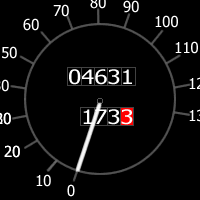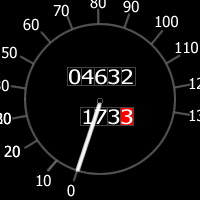#### You may also like### Consecutive Numbers

An investigation involving adding and subtracting sets of consecutive numbers. Lots to find out, lots to explore.### Calendar Capers

Choose any three by three square of dates on a calendar page...### Days and Dates

Investigate how you can work out what day of the week your birthday will be on next year, and the year after...

# How Many Miles to Go?

##### Age 11 to 14Challenge Level

A car's milometer reads $4631$ miles and the trip meter has $173.3$ on it:How many more miles must the car travel before the two numbers contain the same digits in the same order?

Can you find some other starting numbers that will lead to matching pairs?

What is the relationship between two starting numbers that will work?

If the milometer and trip meters had the following exact readings ($4632$ and $173.3$) then the two numbers will not contain the same digits in the same order before the milometer reaches $99999$ miles. Why is this?If the milometer 'loops the clock' back to $00000$ again at $100,000$ miles and the trip meter loops the clock back to $000.0$ at $1000$ miles, will the two numbers ever contain the same digits in the same order?